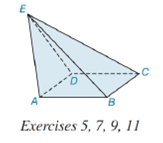Chapter 9.2, Problem 9E### Elementary Geometry for College St...

6th Edition
Daniel C. Alexander + 1 other
ISBN: 9781285195698

#### Solutions

Chapter
Section### Elementary Geometry for College St...

6th Edition
Daniel C. Alexander + 1 other
ISBN: 9781285195698
Textbook Problem
1 views

# Suppose that the lateral faces of the pyramid in Exercise 5 have A A B E = 12 i n 2 , A B C E = 16 i n 2 , A C D E = 12 i n 2 , and A D A E = 10 i n 2 . If each side of the square base measures 4 i n . , find the total surface area of the pyramid.To determine

To find:

The total surface area of the given pyramid.

Explanation

Given:

The figure given below

and base ABCD is a square. The lateral faces of the pyramid measures AABE=12in2, ABCE=16in2, ACDE=12in2, and ADAE=10in2. Each side of the square base measures 4in..

Formula Used:

A pyramid is made by connecting a base to an apex or vertex. The base is flat with straight edges, no curves, hence, a polygon and all other faces are triangles.

A regular pyramid is a pyramid whose base is a regular polygon and whose lateral edges are all congruent.

The lateral faces are given by the triangular faces of the pyramid.

The base face is given by the base polygon.

The total surface area T of a pyramid with lateral area A and base area B is given by

T=L+B.

The area of a square B of side a is given by

B=a2.

Calculation:

From the given figure

The apex is not directly above the center of the base. Hence it is an Oblique Pyramid and the lateral edges are not congruent.

The lateral faces are not congruent. Thus, an irregular pyramid.

The lateral faces are ABE, BCE, CDE, and DAE.

The lateral area L is given by the sum of area of all lateral faces

### Still sussing out bartleby?

Check out a sample textbook solution.

See a sample solution

#### The Solution to Your Study Problems

Bartleby provides explanations to thousands of textbook problems written by our experts, many with advanced degrees!

Get Started